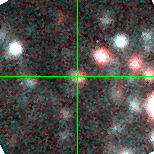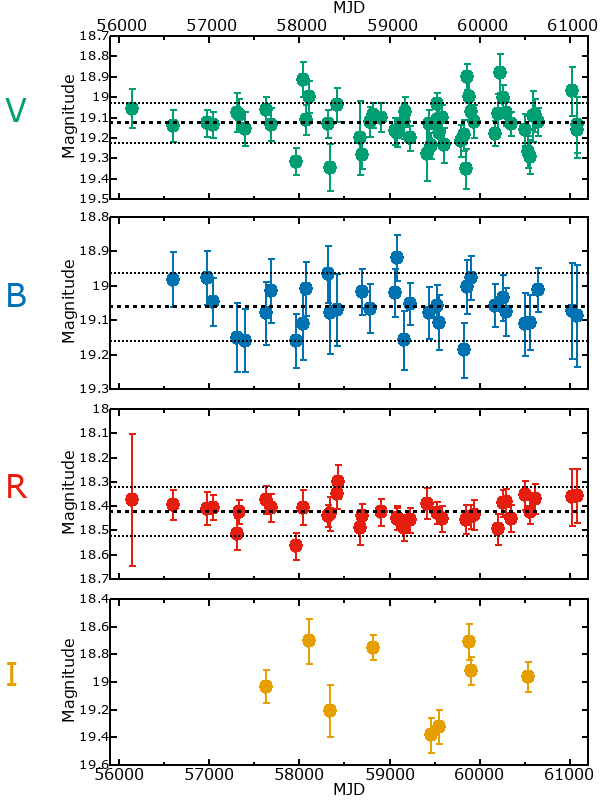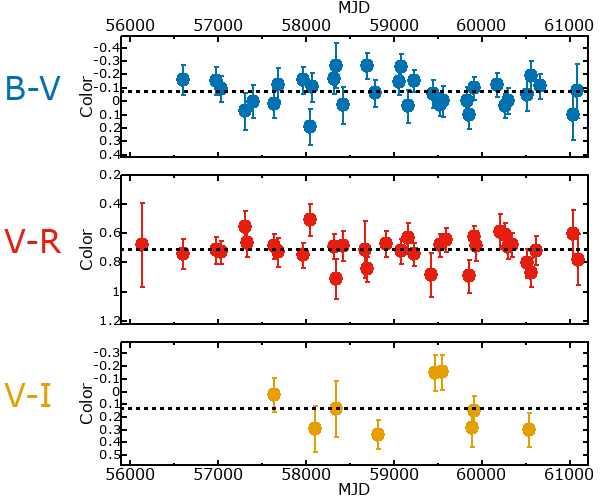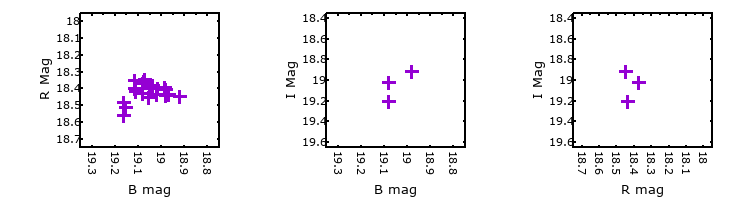#M33-013500.30 (J013500.30+304150.9)

## Target Notes### Classification: B[e]sg

Resolved from two LGGS stars 1 mag fainter 2-3 arcseconds S and E.

## Plots of Brightness and Color## Variability

From the data available in this survey, it is not possible to tell with certainty if this star is variable.

### Correlation factors between bands

If the brightness variation is true variability then there should be a correlation between brightness changes recorded in different filter bands. The following table are the R-squared linear correlation factors calculated for the pairs of bands. The number in parentheses next to the R-squared value is the number of elements used to calculate the correlation factor. The number in square brackets next to the band is the range of magnitudes measured.

V [0.24 mag]B [0.34 mag]R [0.24 mag]
B [0.24 mag]0.01 (19)
R [0.24 mag]0.03 (19)0.41 (15)
I [0.00 mag]0.72 (4)-.-- (2) -.-- (2)

### Magnitude-Magnitude Plots

The plots below are provided to check the values in the table above and also to show any non-linear correlations### I & R Statistics

Welch & Stetson (1993, AJ, 105, 1813) present a method for varible star detection in CCD images from automated surveys. Data from two bands are compared and two statistics are calculated: R and I.

The I-statistic has an expectation value of zero for stars that are NOT variable. A larger non-zero I-value indicates a higher likelihood that the star is variable. The R statistic indicates how the color of the star changes as the variable grows brighter with R = 1.0 being no change in color.

R and I values have only been calculated if there are more than four (4) matched measurements of brightness in each band.
V
(I|R)
B
(I|R)
R
(I|R)
B
(I|R)
0.11 | 1.14
R
(I|R)
0.18 | 1.210.34 | 0.07
I
(I|R)
--- | ------ | --- --- | ----